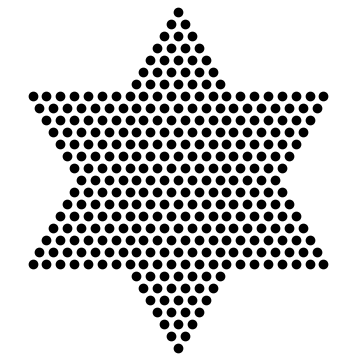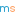# Christmas conundrum #3

Try our nonograms! And remember, not all puzzles have a unique solution…It’s time for the next Chalkdust Christmas Conundrum! But first it’s time to announce the lucky winners of last week’s competition. There were 206 entries, of which 189 were correct. The four randomly selected winners are:

• Josie Neal
• Maureen Monroe
• Antonio S. Macias
• Tom Lock

You will all receive copies of The Indisputable Existence of Santa Claus by Hannah Fry and Thomas Oléron Evans. Hope you enjoy them! The answer to last week’s conundrum is at the bottom of this post.

The prizes up for grabs this week are four copies of The Element in the Room by Helen Arney and Steve Mould. To encourage you to try to win this, we will be publishing a review of it on Thursday.

This week’s conundrum is all about nonograms (AKA griddler, picross, tsunami, or “oh, that puzzle”). If you are unfamiliar with nonograms, we’ve prepared a little seasonal introduction to them. Otherwise, feel free to scroll down and dive straight into the third conundrum.

## An Introduction to Nonograms

A nonogram is a logic puzzle where the object is to fill in squares to create a picture using the numbers around the edge of the puzzle.

Consider the following puzzle.

The first column has a single one above it. This means there will be a single square filled in this column. Similarly for the first row. The second column has $1 \, 2$ written above the column. This means there will be one filled in square followed by a group of two filled in squares (working downwards).  The key point here is that there must be at least one blank square between the single square and the group—however it could be more.

Here is another puzzle to try.

Now that you are familiar with nonograms, it’s time for this week’s conundrum:

## Chalkdust Christmas conundrum #3

The following nonogram puzzle doesn’t have a unique answer: there is more than one pattern of coloured squares that satisfied the clues. How many different solutions are there?

Once you’ve worked out how many possible solutions there are, please enter this into the form below for a chance to win one of four copies of The Element in the Room. The deadline for entries is 12:00 (midday) on Friday 22 December. The winners will be announced on Friday afternoon, when we will be publishing the fourth conundrum and giving you a chance to win a copy of Bletchley Park Brainteasers by Sinclair McKay.

This competition is now closed.

## The solution to conundrum #2

In the second conundrum, we asked you to find the number in the star at the top of three. Stop reading now if you don’t want to know the answer yet.

The two numbers between 14 are a cube number and a triangle number: these must be 8 and 6. Next you can see that 23 is the sum of 8, two times a triangle number and a square number: the triangle and square numbers must be 3 and 9.

Next, call the square number at the bottom left $a$, the square number at the top left $b$, and the triangle number at the top right $c$. Adding upwards, we find that $a+b+45=106$ and $a+141+c=198$; and so $a+b=61$ and $a+c=57$. The only two square numbers that add to 61 are 25 and 36. Therefore $c$ must be 21 or 32, but must be 21 as 32 is not a triangle number. And so $a$ is 36 and $b$ is 25.

Putting all these numbers into the tree gives the top number as 433. This is fitting because 433 is in fact a star number:433 dots arranged to make a star.Eleanor is a maths PhD student at UCL. She is interested in the applications of mathematical modelling to biological and medical problems.
@eleanordoman    + More articles by EleanorMatthew Scroggs is a PhD student at UCL working on finite and boundary element methods. His website, mscroggs.co.uk, is full of maths and now features a video of him completing a level of Pac-Man optimally.
@mscroggsmscroggs.co.uk    + More articles by Matthew